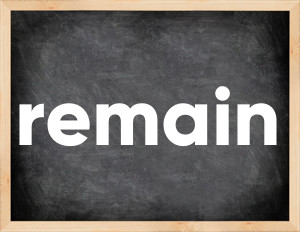# Remain past tenseThe English verb 'remain' is pronounced as [rɪˈmeɪn].
Related to: regular verbs.
3 forms of verb remain: Infinitive (remain), Past Simple - (remained), Past Participle - (remained).

## Here are the past tense forms of the verb remain

👉 Forms of verb remain in future and past simple and past participle.
❓ What is the past tense of remain.

## Remain: Past, Present, and Participle Forms

Base Form Past Simple Past Participle
remain [rɪˈmeɪn]

remained [rɪˈmeɪnd]

remained [rɪˈmeɪnd]

## What are the 2nd and 3rd forms of the verb remain?

🎓 What are the past simple, future simple, present perfect, past perfect, and future perfect forms of the base form (infinitive) 'remain'?

### Learn the three forms of the English verb 'remain'

• the first form (V1) is 'remain' used in present simple and future simple tenses.
• the second form (V2) is 'remained' used in past simple tense.
• the third form (V3) is 'remained' used in present perfect and past perfect tenses.

## What are the past tense and past participle of remain?

The past tense and past participle of remain are: remain in past simple is remained, and past participle is remained.

### What is the past tense of remain?

The past tense of the verb "remain" is "remained", and the past participle is "remained".

### Verb Tenses

Past simple — remain in past simple remained (V2).
Future simple — remain in future simple is remain (will + V1).
Present Perfect — remain in present perfect tense is remained (have/has + V3).
Past Perfect — remain in past perfect tense is remained (had + V3).

### remain regular or irregular verb?

👉 Is 'remain' a regular or irregular verb? The verb 'remain' is regular verb.

## Examples of Verb remain in Sentences

•   Several important problems remain to be settled (Present Simple)
•   Some members of the committee remained skeptical (Past Simple)
•   Real growth rates in most countries have remained quite low (Present Perfect)
•   We decided to remain friends. (Past Simple)
•   These amounts remained payable. (Past Simple)
•   Will remained motionless. (Past Simple)
•   Little will remain after the fire. (Future Simple)
•   Fortunately, the weather remained good. (Past Simple)
•   She remained unspoilt by the money. (Past Simple)
•   She stubbornly remained in the same position. (Past Simple)

Along with remain, words are popular reduce and welcome.

Verbs by letter: , , , , , , , , , , , , , , , , , , , , , , , , .